# Direction Sense Test - Logical Reasoning (MCQ) Questions

Dear Readers, Welcome to Logical Reasoning Direction Sense Test questions and answers with explanation. These Direction Sense Test solved examples with shortcuts and tricks will help you learn and practice for your Placement Test and competitive exams like Bank PO, IBPS PO, SBI PO, RRB PO, RBI Assistant, LIC,SSC, MBA - MAT, XAT, CAT, NMAT, UPSC, NET etc.

After practicing these tricky Direction Sense Test multiple choice questions, you will be exam ready to deal with any objective type questions.

1)   Study the information given below carefully, and answer the question that follow:

On a stage, D, N, A and P are standing as described below facing North.
1) N is 2.5 m to the west of A.
2) K is 4 m to the right of A.
3) D is 6 m to the south of K.
4) P is 9 m to the north of D.

If a boy walks from N, meets A followed by K, D and then P, how many metres has he walked if he has travelled the straight distance all through?

- Published on 15 May 17

a. 15 m
b. 18 m
c. 21.5 m
d. 22.5 m
 Answer  Explanation ANSWER: 21.5 m Explanation: Required distance = NA + AK + KD + DP = 2.5 + 4 + 6 + (6 + 3) = 21.5 m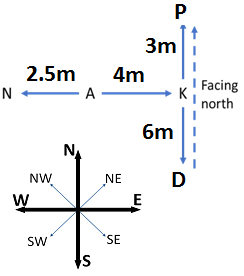6

2)   Study the information given below carefully, and answer the question that follow:
• On a stage, D, N, A and P are standing as described below facing North.
1) N is 2.5 m to the west of A.
2) K is 4 m to the right of A.
3) D is 6 m to the south of K.
4) P is 9 m to the north of D.

Who is to the south-west of P?

- Published on 15 May 17

a. A
b. D
c. K
d. Neither N nor A
 Answer  Explanation ANSWER: A Explanation: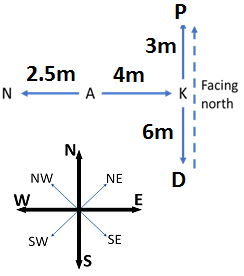A is to the south-west of P.

• A billionaire drove his Ferrari towards North, then turned left and drove 10 km and again turned left and covered 20 km.
• He found himself 10 km West of his starting point.
How far did he drive northward initially?

- Published on 15 May 17

a. 10 km
b. 15 km
c. 20 km
d. 25 km
 Answer  Explanation ANSWER: 20 km Explanation: The billionaire drove 20 km in North direction initially.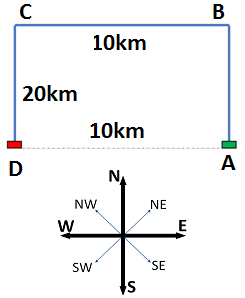• Raees walks 10 m towards East and then turns left and walks 12 m.
• Again, he turns right and walks 18 m.
• Finally, he turns to his right and walks 12 m.
How far is Raees from the starting point?

- Published on 08 May 17

a. 28 m
b. 29 m
c. 30 m
d. 32 m
 Answer  Explanation ANSWER: 28 m Explanation: Required distance = AE = 10 + 18 = 28 m• Raja walked 10 m from A to B in the East.
• Then he turned to the right and walked 3 m.
• Again, he turned to the right and walked 14 m.
How far is Raja from A?

- Published on 08 May 17

a. 8 m
b. 10 m
c. 12 m
d. 14 m
 Answer  Explanation ANSWER: 10 m Explanation:• Some boys are sitting in three rows all facing North such that B is in the middle row. C is just to the right of B but in the same row.
• D is just behind of C while A is in the North of B.
In which direction of A is D?

- Published on 08 May 17

a. South-East
b. South-West
c. North-West
d. North-East
 Answer  Explanation ANSWER: South-East Explanation: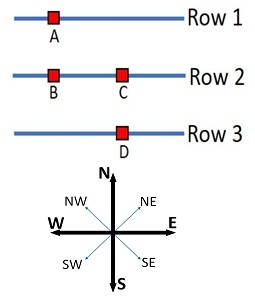• Goku started from his house towards North direction.
• After covering 80 km, he turned towards left and covered a distance of 60 km.
What is the shortest distance now from his house?

- Published on 08 May 17

a. 100 km
b. 110 km
c. 130 km
d. 140 km
 Answer  Explanation ANSWER: 100 km Explanation: Shortest distance = ACAC2 = AB2 + BC2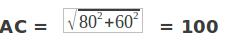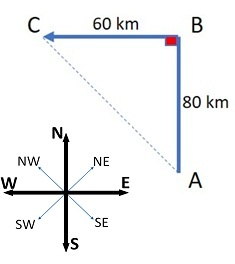• A man walks 10 km toward south and then turns to the right.
• After walking 5 km he turns to the left and walks 10 km.
In which direction is he from the starting position?

- Published on 08 May 17

a. South-East
b. South-West
c. North-West
d. North-East
 Answer  Explanation ANSWER: South-West Explanation: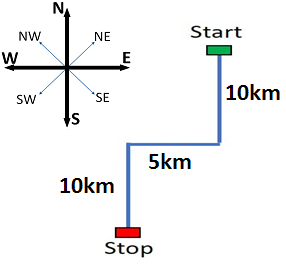9)   One morning, Manish and Ali were talking to each other face to face at a crossing. If Ali's shadow was exactly to the left of Manish, which direction was Manish facing?
- Published on 08 May 17

a. East
b. West
c. South
d. North
 Answer  Explanation ANSWER: North Explanation: The sun rises in the East. Hence, any shadow falls in the West.Ali's shadow was to the left of Manish.Hence, Manish was facing towards North.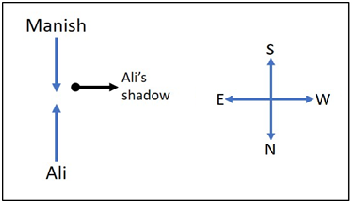10)   Ravindra Jadeja left home and ran 20 km towards South, then turned right and ran 10 km.
He took rest for ten minutes at the same spot and then again turned right and ran 20 km.
After this he turned left and ran 20 km to the cricket ground.

How many kilometers will he have to run back to reach his home?

- Published on 03 May 17

a. 15 km
b. 20 km
c. 25 km
d. 30 km
 Answer  Explanation ANSWER: 30 km Explanation: Required distance AE = 10 + 20 = 30 km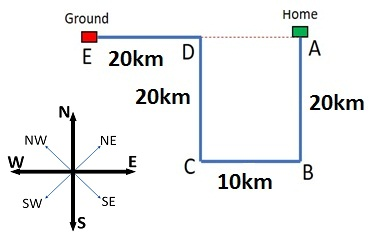1 2 3